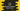# Java program to find all prime numbers from 1 to N## Java program to find all prime numbers from 1 to N:

Java program to find all prime numbers from 1 to N. In this post, we will learn how to find all prime numbers from 1 to N in different ways. The program will take the value of N as an input from the user and it will print all prime numbers from 1 to N.

### How to find if a number is prime or not:

A number is called a prime number if it is greater than 1 and it is divisible only by 1 and the number itself. For example, 2, 3, 5, 7, 11, 13, 17, 19 etc. are prime numbers. Similarly, 4, 6, 8, 9, 10, 12, 14, 15, 16 etc. are not.

To find if a number is prime number or not, we can run a loop from 2 to number/2. If we find any value of the loop that can divide the number, it will not be a prime number. Else, the number will be a prime number.

We can also make one improvement to the above algorithm. Instead of running a loop from 2 to number/2, we can run a loop from 2 to square root of the number. It will also give the same result but the number of iteration will be less with this way.

We need to run another loop from 2 to N, where N is the upper limit of the range. Inside this loop, we will check for each number if it is a prime number or not.

Let’s write down the program using the algorithms we discussed above:

### Method 1: By iterating from 2 to number/2 to check for prime:

With this method, we will write a separate function that will run from 2 to number/2 to check for a prime number.

``````import java.util.Scanner;

class Main {
public static boolean isPrime(int n) {
if (n == 0 || n == 1) return false;

for (int i = 2; i <= n / 2; i++) {
if (n % i == 0) return false;
}
return true;
}

public static void main(String[] args) {
int n;

Scanner sc = new Scanner(System.in);
System.out.println("Enter the value of N: ");
n = sc.nextInt();

System.out.println("Prime numbers between 1 to " + n + " are:");
for (int i = 2; i <= n; i++) {
if (isPrime(i)) {
System.out.print(i + " ");
}
}

}
}``````

Here,

• n is a variable to hold the upper limit or the value of N.
• sc is a Scanner variable to read the user input value. It asks the user to enter the value of N and stores it in n.
• The for loop runs from i = 2 to i = n and for each value of i, it calls isPrime method to check if it is a prime number or not. If yes, it prints the value of i.

• The isPrime method returns false if the number is either 0 or 1.
• It runs a for loop from i = 2 to i = n/2 and for each value of i, it checks if it can divide n perfectly or not. If yes, it returns false.
• Once the loop ends, it returns true.

If you run this program, it will print output as like below:

``````Enter the value of N:
100
Prime numbers between 1 to 100 are:
2 3 5 7 11 13 17 19 23 29 31 37 41 43 47 53 59 61 67 71 73 79 83 89 97``````

### Method 2: By iterating from 2 to square root of number to check for prime:

Another way is to iterate from 2 to square root of the number. As I explained above, it will reduce the number of time the loop iterates. We can use Math.sqrt method to find the square root of a number. This is a predefined function defined in the Math class.

Let’s write down the program:

``````import java.util.Scanner;

class Main {
public static boolean isPrime(int n) {
if (n == 0 || n == 1) return false;

for (int i = 2; i <= Math.sqrt(n); i++) {
if (n % i == 0) return false;
}
return true;
}

public static void main(String[] args) {
int n;

Scanner sc = new Scanner(System.in);
System.out.println("Enter the value of N: ");
n = sc.nextInt();

System.out.println("Prime numbers between 1 to " + n + " are:");
for (int i = 2; i <= n; i++) {
if (isPrime(i)) {
System.out.print(i + " ");
}
}

}
}``````

It is almost similar to the first program. The only difference is that it iterates from 2 to Math.sqrt of n.

If you run this program, it will print similar output.

### Method 3: By using a while loop:

We can also use a while loop instead of a for loop to write the same program. Let’s write it with a while loop:

``````import java.util.Scanner;

class Main {
public static boolean isPrime(int n) {
if (n == 0 || n == 1) return false;
int i = 2;

while (i <= Math.sqrt(n)) {
if (n % i == 0) return false;
i++;
}
return true;
}

public static void main(String[] args) {
int n, i = 2;

Scanner sc = new Scanner(System.in);
System.out.println("Enter the value of N: ");
n = sc.nextInt();

System.out.println("Prime numbers between 1 to " + n + " are:");
while (i <= n) {
if (isPrime(i)) {
System.out.print(i + " ");
}
i++;
}

}
}``````

Both for loops are replaced with while loops. It will give similar result.